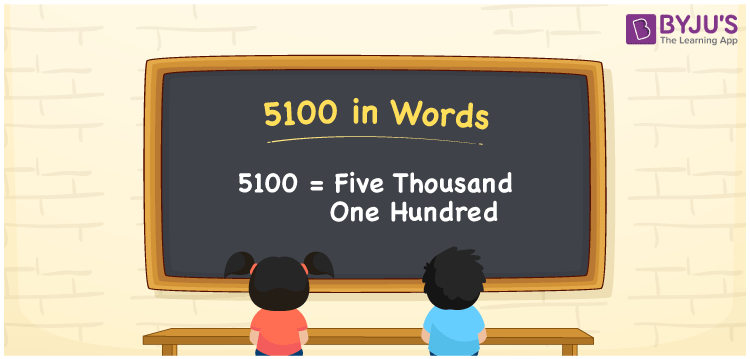# 5100 in Words

5100 in words is expressed as Five Thousand One Hundred. For example, if you saved Rs. 5100 this month, you can say, “I saved Rupees Five Thousand One Hundred this month”. 5100 is a cardinal number as it denotes a certain amount. Place value charts enable students to determine the numerical name of any number. Hence, using a place value chart is vital while converting the numbers to words. This article provides detailed information about how to convert the number 5100 to words as well as how to spell in English words.

 5100 in words Five Thousand One Hundred Five Thousand One Hundred in numerical form 5100

## 5100 in English Words

The English language is commonly used in the education field. Thus, we write the numbers in words using the letters of the English alphabet. Therefore, we can express the number 5100 in English as Five Thousand One Hundred.## How to Write 5100 in Words?

Determine the place value of each digit in 5100 before conversion to word form. The following table shows the place value chart for 5100 along with its expanded form.

 Thousands Hundreds Tens Ones 5 1 0 0

Therefore, we can write the expanded form as:

5 x Thousand + 1 x Hundred + 0 x Ten + 0 x One

= 5 x 1000 + 1 x 100 + 0 x 10 + 0 x 1

= 5000 + 100 + 0 + 0

= 5000 + 100

= 5100

= Five Thousand One Hundred

Therefore, 5100 in words is written as Five Thousand One Hundred

Interesting way of writing 5100 in words

5 = Five

51 = Fifty-One

510 = Five Hundred and Ten

5100 = Five Thousand One Hundred

Thus, the word form of the number 5100 is Five Thousand One Hundred

5100 is a natural number that precedes 5101 and precedes 5099

• 5100 in words – Five Thousand One Hundred
• Is 5100 an odd number? – No
• Is 5100 an even number? – Yes
• Is 5100 a perfect square number? – No
• Is 5100 a perfect cube number? – No
• Is 5100 a prime number? – No
• Is 5100 a composite number? – Yes

## Frequently Asked Questions on 5100 in Words

Q1

### How do you write 5100 in words?

The number 5100 in words can be written as Five Thousand One Hundred.
Q2

### How to write Rs. 5100 on a cheque?

On a cheque, Rs. 5100 in words is written as Five Thousand One Hundred rupees only.
Q3

### Is 5100 a composite number?

Yes, the number 5100 is a composite number.